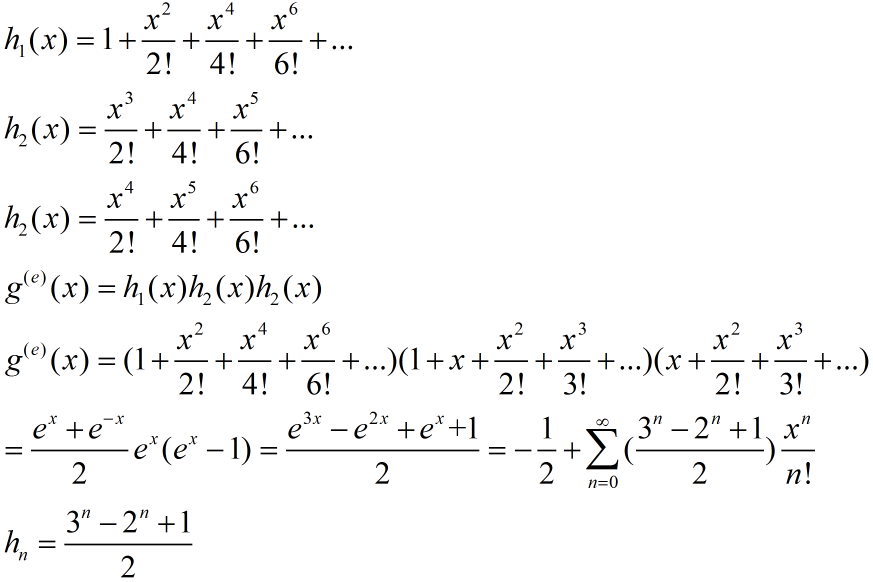# 组合数学之三 —— 生成函数

## 知识铺垫

#### 一 . 算术数列

An=A0+nq
Sn=( n-1 ) * A0+( q * n *（n+1）)/2

#### 二 . 几何数列

An=A0*q^n
Sn=A0*( q^n-1 ) / ( q-1 ) —> (q!=1)

#### 三 . 斐波那契数列

① f(n)=f(n-1)+f(n-2) (n>=2)
② f(0)=0，f(1)=1
③ Sn=f(0)+f(1)+f(2)+…+f(n)=f(n+2)-1

S(n+1)
=f(0)+f(1)+f(2)+…+f(n)+f(n+1)
=[f(0)+f(1)+f(2)+…+f(n)]+f(n+1)
=f(n+2)-1+f(n+1)
=f(n+3)-1

④ 斐波那契数f(n)是偶数当且仅当n能被3整除
⑤ 斐波那契数列通项公式：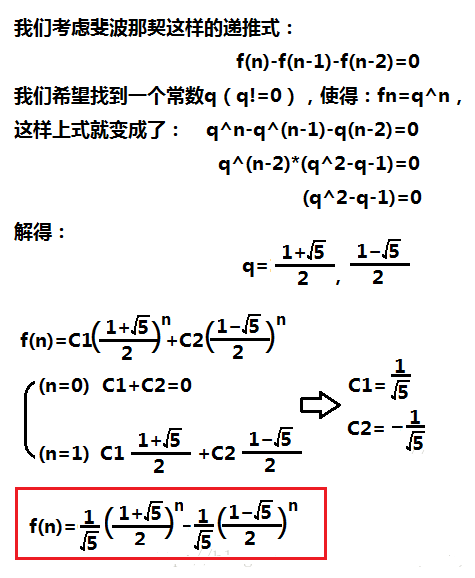⑥ 在帕斯卡三角形从左上到右下的对角线上的二项式系数的和是斐波那契数列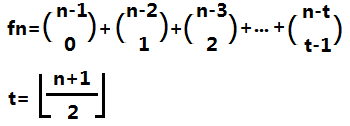## 生成函数

h0 , h1 , h2 , … , hn , …

ta的生成函数定义为无穷函数：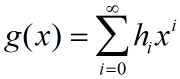#### ∞ 例一

g(x)=1+x+x^2+…+x^n+….==1/(1-x)

#### ∞ 例二

C(m,0)，C(m,1)，C(m,2)，…，C(m,m)

g(x)=C(m,0)+C(m,1)x+C(m,2)x^2+…+C(m,m)x^m

#### ∞ 例三（重点）

（建议多读几遍题）

hn=C(n+k-1,k-1)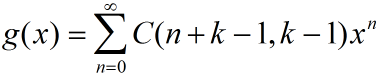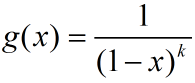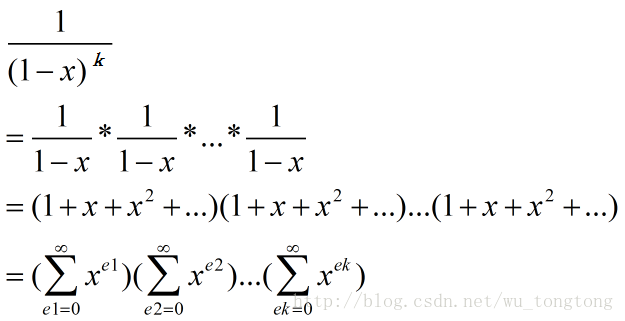$\left(x+y{\right)}^{n}=\sum _{k=0}^{n}C\left(n,k\right){x}^{k}{y}^{n-k}$$(x+y)^n=\sum_{k=0}^{n}C(n,k)x^ky^{n-k}$
$\left(1+x{\right)}^{n}=\sum _{k=0}^{n}C\left(n,k\right){x}^{k}$$(1+x)^n=\sum_{k=0}^{n}C(n,k)x^k$

$\left(1+x{\right)}^{n}=\sum _{k=0}^{\infty }C\left(n,k\right){x}^{k}$

$\left(1+x{\right)}^{-n}=\sum _{k=0}^{\infty }C\left(-n,k\right){x}^{k}=\sum _{k=0}^{\infty }\left(-1{\right)}^{k}C\left(n+k-1,k\right){x}^{k}$

$\left(1+\left(-x\right){\right)}^{-n}=\sum _{k=0}^{\infty }\left(-1{\right)}^{k}C\left(n+k-1,k\right)\left(-x{\right)}^{k}$

$=\sum _{k=0}^{\infty }\left(-1{\right)}^{k}C\left(n+k-1,k\right)\left(-1{\right)}^{k}{x}^{k}=\sum _{k=0}^{\infty }C\left(n+k-1,k\right){x}^{k}$

$\left(1-x{\right)}^{-n}=\sum _{k=0}^{\infty }C\left(n+k-1,k\right){x}^{k}=\sum _{k=0}^{\infty }C\left(n+k-1,n-1\right){x}^{k}$

$n$$n$$k$$k$交换一下：

${\left(1-x\right)}^{-k}=\sum _{n=0}^{\infty }C\left(k+n-1,k-1\right){x}^{n}$

x^e1*x^e2*…x^ek=x^n
e1+e2+…+ek=n

#### ∞ 例四

(1+x+x^2+x^3+x^4+x^5)(1+x+x^2)(1+x+x^2+x^3+x^4)

#### ∞ 例五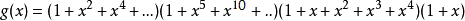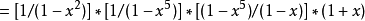#### ∞ 例六

f1=3*e1 , f2=4*e2 , f3=2*e3 , f4=5*e4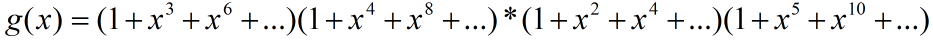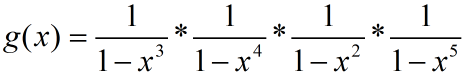#### 我们在这里提出一个定理：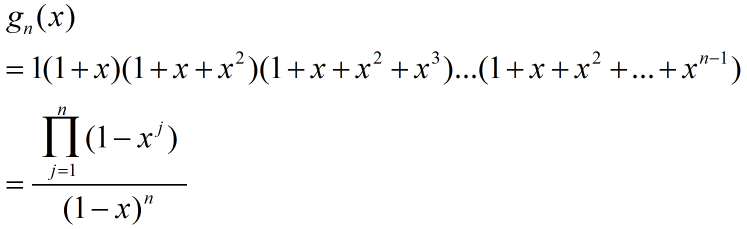## 指数生成函数

{1，x，x^2，x^3，…}

{1，x，x^2/2！，x^3/3！，x^4/4！，…}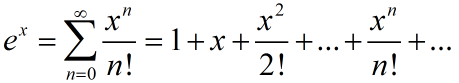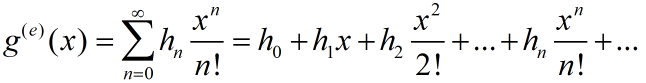#### 常用指数生成函数的闭形式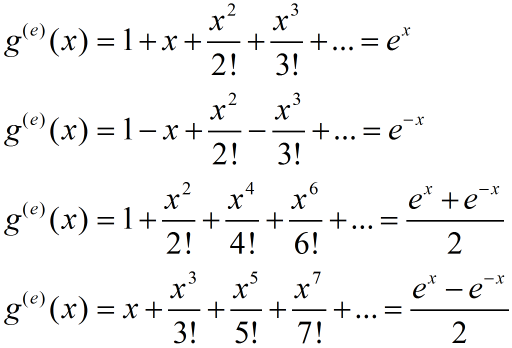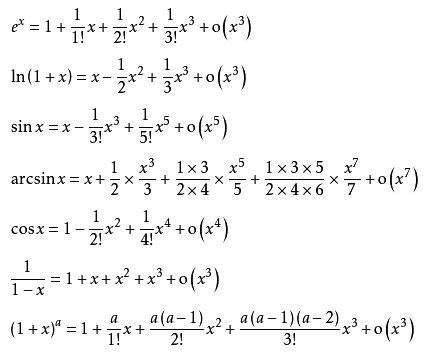#### ∞ 例一

P(n,0)，P(n,1)，P(n,2)，…，P(n,n)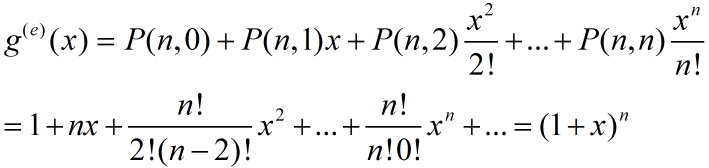C(n,0)，C(n,1)，C(n,2)，…，C(n,n)

#### ∞ 例二

1，a，a^2，a^3，…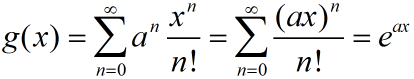#### 我们在这里再提出一个定理：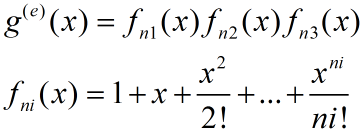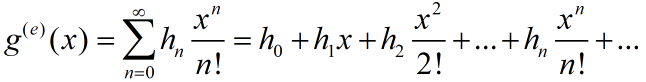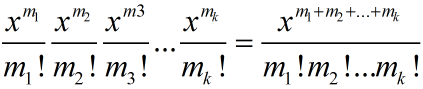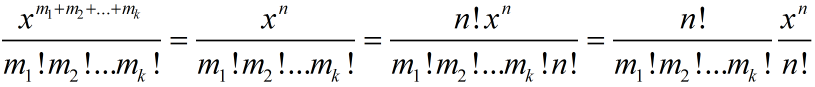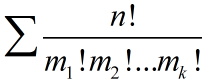0<=m1<=n1，0<=m2<=n2，0<=m3<=n3，0<=mk<=nk
m1+m2+m3+…+mk=n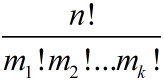#### ∞ 例三

hi(x)表示对应因子的指数生成函数：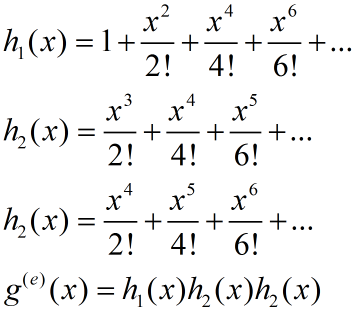#### ∞ 例四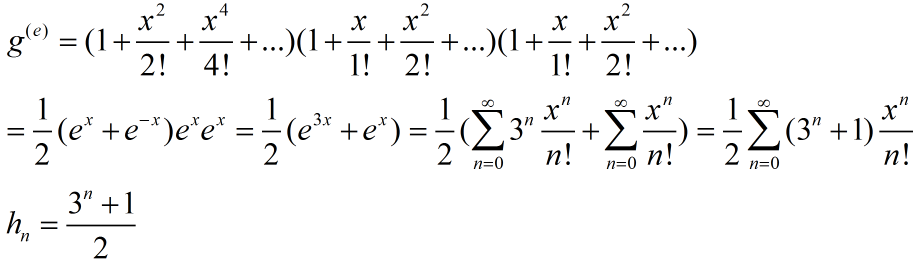#### ∞ 例五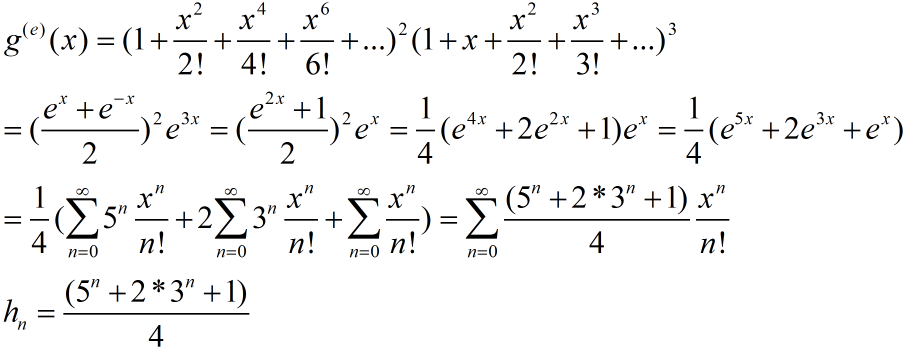#### ∞ 例六